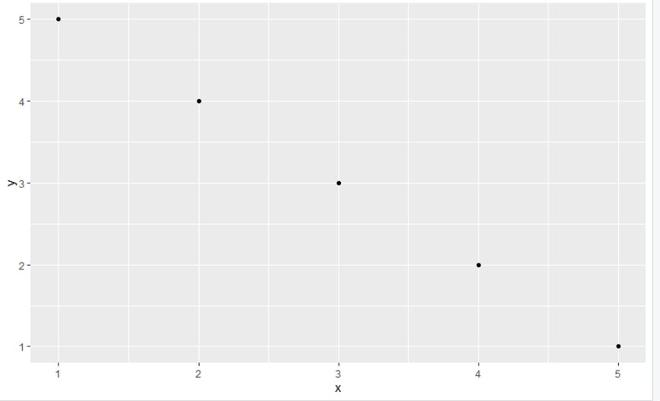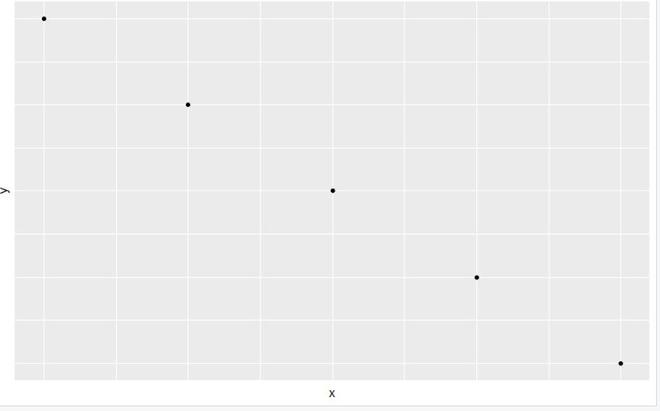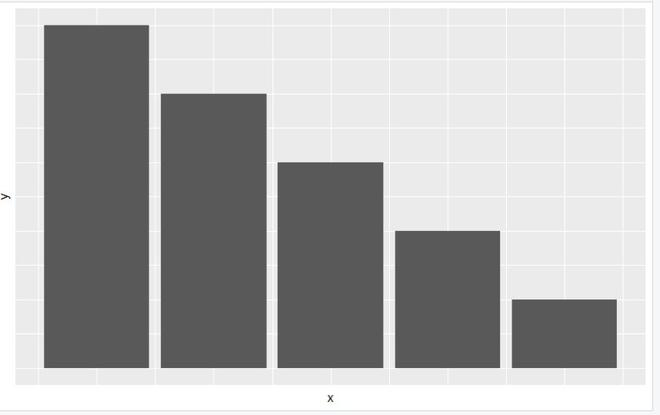# Remove Axis Labels using ggplot2 in R

• Last Updated : 06 Jun, 2021

In this article, we are going to see how to remove axis labels of the ggplot2 plot in the R programming language.

We will use theme() function from ggplot2 package. In this approach to remove the ggplot2 plot labels, the user first has to import and load the ggplot2 package in the R console, which is a prerequisite for this approach, then the user has to call the theme() function which is the function of the ggplot2 package and further needs to pass the element_blank() as its parameters, which will be helping to remove the ggplot2 plots labels as blank in R programming language.

theme() function: Use of this function is a powerful way to customize the non-data components of your plots: i.e. titles, labels, fonts, background, gridlines, and legends. This function can also be used to give plots a consistent customized look.

In this example, we will be showing that how the plot looks without removing its labels.

## R

 `library``(``"ggplot2"``)    ` `gfg_data <- ``data.frame``(x = ``c``(1,2,3,4,5), ` `                       ``y = ``c``(5,4,3,2,1)) ` `gfg_plot <- ``ggplot``(gfg_data, ``aes``(x,y)) +   ` `  ``geom_point``() ` `gfg_plot`

Output:Example 1: In this example, we will be removing the label of the ggplot2  scatter plot of five data point using the theme() function of the ggplot2 package in the R programming language.

## R

 `library``(``"ggplot2"``)    ` `gfg_data<-``data.frame``(x = ``c``(1,2,3,4,5), ` `                     ``y = ``c``(5,4,3,2,1)) ` `gfg_plot <- ``ggplot``(gfg_data, ``aes``(x,y)) +   ` `  ``geom_point``() ` `gfg_plot + ` `  ``theme``(axis.text.x = ``element_blank``(), ` `        ``axis.ticks.x = ``element_blank``(), ` `        ``axis.text.y = ``element_blank``(), ` `        ``axis.ticks.y = ``element_blank``())`

Output:Example 2: In this example, we will be removing the labels of the ggplot2 bar plot using the theme() function from the ggplot2 package in the R programming language.

## R

 `library``(``"ggplot2"``)    ` `gfg_data<-``data.frame``(x = ``c``(1,2,3,4,5), ` `                     ``y = ``c``(5,4,3,2,1)) ` `p<-``ggplot``(data=gfg_data, ``aes``(x, y)) + ` `  ``geom_bar``(stat=``"identity"``) ` `p+ ` `  ``theme``(axis.text.x = ``element_blank``(), ` `        ``axis.ticks.x = ``element_blank``(), ` `        ``axis.text.y = ``element_blank``(), ` `        ``axis.ticks.y = ``element_blank``()) `

Output:My Personal Notes arrow_drop_up
Recommended Articles
Page :